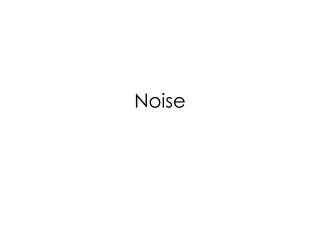DownloadDownload PresentationNoise

# Noise

Télécharger la présentation## Noise

- - - - - - - - - - - - - - - - - - - - - - - - - - - E N D - - - - - - - - - - - - - - - - - - - - - - - - - - -
##### Presentation Transcript

1. Noise

2. Resistor Thermal Noise

3. Example Vnr1sqr=2.3288 x 10-19 Vnr3sqr=7.7625 x 10-20 Vnoutsqr=3.1050 x10-19

4. Analytical Versus Simulation

5. Popular Interview Question

6. Noise Spectrum Shaping by a Low Pass Filter As R increases, 4kTR increases, but the bandwidth decreases. Therefore, the bandwidth is constant. Pn,out can only be decreased by increasing C.

7. Alternative Representation of Resistor Thermal Noise

8. MOSFETS (Typically 2/3, not to be confused with body effect coefficient)

9. as a function of length

10. Noise Voltage Generated Per Device VDS=0.6 I1=100 uA

11. Flicker Noise

12. Flicker Noise Model The flicker noise is modeled as a voltage source in series with the gate: The trap-and-release phenomenon associated with the dangling bond occurs at low frequencies more often. Device area can be increased to decrease noise due to flicker noise.

13. Corner Frequency • Definition: the frequency at which the thermal noise equals the flicker noise.

14. Corner Frequency (fco)

15. fco as a function of length

16. Representation of Noise in Circuits • Output noise • Input noise • Voltage noise source • Current noise source

17. Output Noise

18. Problem of Output Noise • Output noise depends on the gain of the amplifier, for example.

19. Input-Referred Noise Voltage Problem: only valid for when source impedance is low.

20. Input Voltage Calculation

21. Calculation of Input-Referred Noise Low source impedance High source impedance

22. Input Current More significant at High frequencies!

23. Noise in Single Stage Amplifier • Equivalent CS Stages • CS • CG • SF • Cascode • Differential Pairs

24. Equivalent CS Stages

25. Common Source Amplifier The transconductance of M1 must be maximized in order to minimize input-referred noise.

26. Input Referred Thermal Noise Voltage M2 acts as the current source. The gm of M2 should be minimized. M1 acts as the amplifier. The gm of M1 should be maximized.

27. Noise Simulation Thermal noise 3.758 nV/sqrt(Hz) Av=28.711

28. Noise Simulation 3.126 nV/sqrt(Hz) Av=33.42

29. Comparison (simulated input-referred thermal noise) Av=33.42

30. Flicker Noise Dominated by Flicker noise Dominated by Thermal noise

31. Common Gate Amplifier • Need to consider • Input referred voltage source • Input referred current source

32. Gain of CG If RS=0 and channel length modulation is ignored, Av is

33. Input-Referred Voltage Source

34. Input-Referred Current Source Does not produce a current to the output

35. Input-Referred Thermal Noise

36. Input-Referred Flicker Noise

37. Design Example Design criteria: gm/ID=5 for M0, M2, M3 and M4. gm/ID=20 for M1. I1=10 uA I2=10 uA I(M1)=40 uA

38. Source Follower with a NMOS CS Load (Review)

39. Source Followers High Input impedance, noise current source is negligible.

40. Cascode Stage (At Low Frequencies)

41. Cascode Stage(At High Frequencies)

42. Differential Pair (negligible)

43. Analysis